Guide to Constraint Programming © Roman Barták, 1998 Contents Prev Up Next

# Algorithms for Solving Constraint Hierarchies

[Simple] [DeltaStar] [DeltaBlue] [SkyBlue] [Indigo] [Houria] [IHCS] [Projection]

A important aspect of constraint hierarchies is that there are efficient satisfaction algorithms proposed to solve them. In this section we overview some of the most popular algorithms for solving constraint hierarchies. We can categorize these algorithms into the following two general approaches:

1. the refining method and
2. the local propagation.

###Refining Algorithms

The refining algorithms first satisfy the constraints on the strongest level of the hierarchy, and then, the constraints on the weaker levels successively. It is a straightforward method for solving constraint hierarchies as it follows directly the definition of the solution of constraint hierarchy, in particular, the property of respecting the hierarchy. Consequently, the refining algorithms can be used to solve all constraint hierarchies, i.e., all type of constraints, using arbitrary comparator.

The disadvantage of refining method is that it has to recompute the solution from scratch everytime a constraint is added or retracted.

#### Simple Algorithm M. Wilson, A. Borning: Hierarchical Constraint Logic Programming, TR 93-01-02a, Department of Computer Science and Engineering, University of Washington, May 1993

The simple algorithm for solving constraint hierarchies performs a recursive search for answers representing locally-predicate-better solutions. It uses underlying "flat" constraint solver to solve individual constraints, i.e., to test consistnency of the system of constraints. The solution is accumulated as a set Answer of satisfiable constraints. Alternative solutions can be found by choosing constraints from Level in a different order.

#### Simple Algorithm for Solving Constraint Hierarchies

```procedure Solve(H: constraint hierarchy)
Untried <-H;
while Untried is not empty do
s <- the strongest level of the constraints in Untried;
Level <- delete constraints with level s from Untried;
while Level is not empty do
c <- delete a constraint from Level; % different order = alternative solutions
if c is compatible with Answer then  % call to flat constraint solver
endif
endwhile
endwhile
end Solve```

You can find implementation of extended version of the simple constraint hierarchy solver for various predicate comparators (even global ones) at my Projects pages.

#### DeltaStar M. Wilson, A. Borning: Hierarchical Constraint Logic Programming, TR 93-01-02a, Department of Computer Science and Engineering, University of Washington, May 1993

While the simple algorithm described above can solve constraint hierarchies using predicate comparators only, the DeltaStar algorithms is able to use metric comparators as well. Again, it uses underlying flat constraint solver to solve constraints, but now it requires the flat constraint solver to provide the procedure

`filter(S:Solution, C:Set of constraints) -> Solution,`

that given an existing solution S returns the subset of S that minimizes the error in satisfying the set of constraints C (the implementation of this routine effectively defines the comparator). In addition, the flat solver should provide other entries for efficiently determing if a new constraint is compatible with a current solution, and for quickly adding a constraint to a current solution, given a guarantee that the constraint is compatible.

#### DeltaStar

```procedure DeltaStar(H: constraint hierarchy)
i <- 1;
Solution <- solution of required constraints from H
while not unique Solution and i<number of levels do
Solution <- filter(Solution,Hi);    % Hi is i-th level in H
i++;
endwhile
return Solution;
end DeltaStar```

DeltaStar uses Simplex algorithm as the underlying flat constraint solver, and therefore it requires some transformation techniques to be applied to the constraints before they can be solved using Simplex.

The following figure illustrates the idea behind the DeltaStar Algorithm.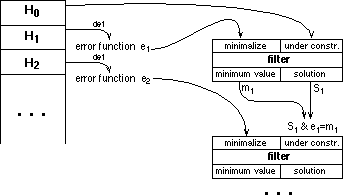###Local Propagation Algorithms

The local propagation algorithms gradually solve constraint hierarchies by repeatedly selecting uniquely satisfiable constraints. In this technique, a single constraint is used to determine the value for a variable. Once this variable's value is known, the system may be able to use another constraint to find a value for another variable, and so forth. This straightforward execution phase is paid off by a foregoing planning phase that chooses the order of constraints to satisfy.

To support local propagation, the object representing a constraint includes one or more pieces of code (methods). Method is a function whose arguments are input variables and that caculates a value for an output variable(s) that will satisfy the constraint (for example, constraint A+B=C, in general, includes three methods C<-A+B, A<-C-B, B<-C-A). Local propagation algorithms select one (or none) method for each constraint and determine the appropriate order of methods to solve the constraint hierarchy.

The advantage of this approach is that when a variable is repeatedly updated, e.g., by user operation, it can easily evaluate only the necessary constraints to get a new solution.

Local propagation is also restricted in some ways:

1. most local propagation algorithms solve equality constraints only,
2. they use locally-predicate better comparator or its variant only,
3. they are not able to solve "cycles" of constraints (without additional gadgets),
4. they cannot find multiple solutions due to uniqueness.

#### DeltaBlue M. Sannella, B. Freeman-Benson, J. Maloney, A. Borning: Multi-way versus One-way Constraints in User Interfaces: Experience with the DeltaBlue Algorithm, TR 92-07-05

DeltaBlue is a typical representative of local propagation algorithms. It solves multi-way (allows more methods for a constraint) constraints with one-output variable. DeltaBlue stores the current solution in the form of a solution graph, which describes how to recompute values for variables in order to satisfy all the satisfiable constraints. The following figure shows such solution graphs: each node in the graph represents a variable, the arcs represent constraints, labeled with their strengths. Arrows on the arcs show which methods are used, while dotted arcs indicate constraints that are unsatisfied.DeltaBlue supports separate planning and execution stages. Given a constraint graph, the algorithm can be used to find a plan for re-satisfying the constraints. During the planning stage, DeltaBlue constructs incrementally the solution graph by adding constraints to the graph. The key idea behind DeltaBlue is to associate extra information, walkabout strength, with the constrained variables so that the solution graph can be updated incrementally when a constraint is added or removed without examining, on the average, more than a small fraction of the entire constraint hierarchy. Walkabout strength is the weaker of the stregth of the constraint currently determining the variable and the weakest walkabout strength among all other potential output of this constraint (if the variable is not detemined by any constraint, then the walkabout strength is weakest).

#### DeltaBlue

```procedure AddConstraint(c: constraint)
select the potential output variable V of c with the weakest walkabout strength
if walkabout strength of V is weaker than strength of c then
c'<- the constraint currently determing the variable V;
make c' unsatisfied;
select the method determing V in c;
recompute walkabout strengths of downstream variables;
endif

The following example animates the process of adding a strong constraint to the constraint graph. Five variables are linked in a chain by required equality constraints, and a weak constraint has been added to the rightmost variable. Nodes are labeled by walkabout stregths.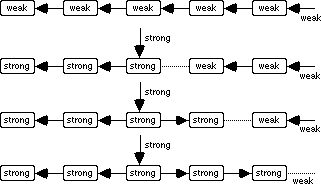DeltaBlue has two limitations: cycles of constraints are prohibited, and the procedures (methods) used to satisfy a constraint can only have a single output.

#### SkyBlue M. Sannella: The SkyBlue Constraint Solver, TR 92-07-02, Department of Computer Science and Engineering, University of Washington, February 1993

SkyBlue is a successor to the DetlaBlue algorithm, which relaxes the restrictions of DeltaBlue, i.e., allowing cycles of constraints to be constructed (although SkyBlue may not be able to satisfy all of the constraints in a cycle) and supporting multi-output methods.

SkyBlue utilizes the same ideas as the DeltaBlue algorithm. Again, it incrementally constructs the constraint network (now called a method graph) and uses the generalized notion of walkabout strength to recompute only the small fraction of the graph after adding or removing a constraint.

#### Indigo A. Borning, R. Anderson, B. Freeman-Benson: The Indigo Algorithm, TR 96-05-01, Department of Computer Science and Engineering, University of Washington, July 1996

Indigo is an efficient local propagation algorithm for satisfying acyclic constraint hierarchies, including inequality constraints, using locally-metric-better comparator. The key idea in Indigo is that it propagates lower and upper bounds on variables (i.e. intervals), rather than specific values. The constraints are processed from strongest to weakest, tightening the bounds on variables.

In contrast to DeltaBlue and SkyBlue, in Indigo, each constraint has a collection of bounds propagation methods. Thus, the A+B=C constraint has three bounds propagation methods, which tighten the bounds on A, B, and C respectively. If we have previously tightened the bounds on say C, when we process the constraint A+B=C we may then need to tighten the bounds on both A and B. This is a sharp contrast to the behaviour of standard local propagation algorithms, in which to satisfy a constraint a single method is executed (and hence a single variable changed). Also, tightening the bounds on constraint's variables may cause the bounds on other variables to be tightened, rippling out to further variables. To implement this, the algorithm keeps a queue of constraints to be checked.

#### Indigo

```procedure Indigo(H: constraint hierarchy)
all_constraints <- list of constraints in H, strongest first;
all_variables <- empty;
active_constraints <- empty;
for each v in all_variables do
initialize v.bounds to unbounded;
endfor

for current_constraint in all_constraints do
tigh_variables <- empty;
queue <- empty;
queue <- queue + current_constraint;
while queue not empty do
cn <- queue.front;
tighten_bounds(cn,queue,tight_variables,active_constraints);
check_constraint(cn,active_constraints);
queue.dequeue;
endwhile
endfor
end Indigo```

The following tables illustrate the process of bounds propagation in Indigo:

 Constraint Hierarchy: ```c1: required a>=10 c2: required b>=20 c3: required a+b=c c4: required c+25=d c5: strong d<=100``` ```c6: medium a=50 c7: weak a=5 c8: weak b=5 c9: weak c=100 c10: weak d=200```

 action a b c d note (-inf,inf) (-inf,inf) (-inf,inf) (-inf,inf) initial bounds add c1 [10,inf) (-inf,inf) (-inf,inf) (-inf,inf) add c2 [10,inf) [20,inf) (-inf,inf) (-inf,inf) add c3 [10,inf) [20,inf) [30,inf) (-inf,inf) add c4 [10,inf) [20,inf) [30,inf) [55,inf) add c5 [10,inf) [20,inf) [30,inf) [55,100] [10,inf) [20,inf) [30,75] [55,100] propagate bounds using c4 [10,55] [20,65] [30,75] [55,100] propagate bounds using c3 add c6 [50,50] [20,65] [30,75] [55,100] [50,50] [20,25] [70,75] [55,100] propagate bounds using c3 [50,50] [20,25] [70,75] [95,100] propagate bounds using c4 add c7 [50,50] [20,25] [70,75] [95,100] c7 is unsatisfied add c8 [50,50] [20,20] [70,75] [95,100] c8 is unsatisfied but its error is minimized [50,50] [20,20] [70,70] [95,100] propagate bounds using c3 [50,50] [20,20] [70,70] [95,95] propagate bounds using c4 add c9 [50,50] [20,20] [70,70] [95,95] c9 is unsatisfied add c10 [50,50] [20,20] [70,70] [95,95] c10 is unsatisfied

#### Houria III M. Bouzoubaa, B. Neveu, G. Hasle: Houria III: Solver for Hierarchical System, Planning of Lexicographic Weight Sum Better Graph for Functional Constraints, in: 5th INFORMS Computer Science Technical Section Conference on Computer Science and Operations Research, Dallas, January 1996

Houria III is an incremental local propagation algorithm for solving constraint hierarchies using globally-better comparators. Similarly to SkyBlue, the Houria III algorithm constructs method graphs that are used to propagate values through constraints. But, Houria III constructs "all" possible method graphs and selects those graphs that satisfy best the constraints. It starts with graphs for required constraints and than it adds incrementally soft constraints to these graphs. To decrease number of maintained graphs, Houria III uses only those partial graphs that can become the solution graphs after adding the constraint.

#### Houria III

```procedure Houria(H: constraint hierarchy)
graphs <- all method graphs for required constraints in H;
queue <- soft (non-required) constraints in H
while queue not empty do
c <- delete the strongest constraint from queue;
graphs <- add c to graphs that can become solution graphs;
endwhile
end Houria```

The following figures animate the process of solving constraint hierarchy using the Houria III algorithm.

 Constraint Hierarchy: ```required A+B=C strong C=5 (0.5) strong A=3 (0.8) strong B=3 (0.8)```

1) alternative method graphs for required constraint (squares = constraints, circles = variables)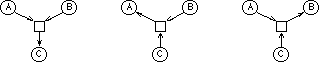2) add methods for the constraint strong C=5; numbers indicate the order of graphs usign weighted-sum-better comparator, dotted arcs indicate unsatisfied constraint3) add methods for the constraint strong A=3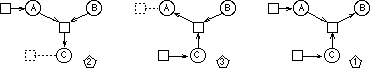4) add methods for the constraint strong B=3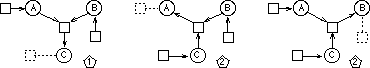###Others

#### Incremental Hierarchical Constraint Solver (IHCS) F. Menezes, P. Barahoma, P. Codognet: An Incremental Hierarchical Constraint Solver, in: Proceedings of PPCP93, pp. 190-199, Newport, 1993

Incremental Hierarchical Constraint Solver (IHCS) is an incremental algorithm for solving constraint hierarchies over finite domains using localy-predicate-better comparator. It is based on idea of transforming initial configuration corresponding to the constraint hierarchy to the solution configuration. A configuration of the hierarchy H is a triple AS•RS•US (AS - active store, RS - relaxed store, US - unexplored store) such that the union of AS, RS and US is equal to H.

The algorithm starts with the configuration 0•0•H and succussively moves constraints from US (initially H) to AS (initially empty) using so called forward rule. As soon as any conflict appears (consistency techniques are used to detect conflicts among constraints), the backward rule is called to change the configuration by switching some constraints between sets AS, RS and US. The algorithm stops as soon as the configuration is AS•RS•0.

#### IHCS

```procedure IHCS(H: constraint hierarchy)
AS•RS•US <- 0•0•H;
while US not empty do
apply forward rule to AS•RS•US, i.e., move c from US to AS
if conflict in AS then
apply backward rule to AS•RS•US;
endif
endwhile
end IHCS```

The following tables illustrate the process of applying forward and backward rules to solve the constraint hierarchy (the initial domains of variables X and Y are [1..10]):

 Constraint Hierarchy: ```c1: strong X+Y=15 c2: strong 3X-Y<5 c3: weak X>Y+1 c4: weak X<7 ```

 action configuration D(X) D(Y) rule add c1 `{ }•{ }•{c1}` 1..10 1..10 fw `{c1 }•{ }•{ }` 5..10 5..10 add c2 `{c1 }•{ }•{c2}` 5..10 5..10 fw `{c1,c2 }•{ }•{ }` - - bw `{c1 }•{c2 }•{ }` 5..10 5..10 add c3 `{c1 }•{c2 }•{c3}` 5..10 5..10 fw `{c1,c3 }•{c2 }•{ }` 7..10 5..8 add c4 `{c1,c3 }•{c2 }•{c4}` 7..10 5..8 fw `{c1,c3,c4}•{c2 }•{ }` - - bw `{c1,c3 }•{c2,c4}•{ }` 7..10 5..8

#### Projection Algorithm W. Harvey, P.J. Stuckey, A. Borning: Compiling Constraint Solving using Projection, in: Proceedings of CP97, Austria, October/November 1997

The Indigo algorithm is able to solve acyclic equality and disequality constraints but as soon as the cycles appear, Indigo fails. Therefore another algorithm based on projection was proposed to solve arbitrary sets of linear equality and disequality constraints using locally-error-better comparator. This algorithm successively eliminates variables using either Gaussian or Fourier elimination.

First, for each variable x in vars (C), the set C of constraints is partitioned in the following way:

• C(0,x): constraints in C that do not contain x,
• C(=,x): equations in C containing x,
• C(+,x): inequalities in C that are equivalent to an inequality of the form x<=e,
• C(-,x): inequalities in C that are equivalent to an inequality of the form e<=x.

Then, the projection algorithm shown bellow eliminates a variable x from the constraint set C.

#### Projection Algorithm

```procedure project(C: set of constraints, x: variable)
if exists c in C(=,x) where c is x=e then
D <- C-{c} with every occurence of x replaced by e;
else
D <- C(0,x);
foreach c in C(+,x) where c is x<=e+ do
foreach c in C(-,x) where c is e-<=x do
D <- D union {e-<=e+};
endfor
endfor
endif
return D;
end project```

Fourier elimination steps in the projection algorithm tend to produce a large number of redundant constraints which can be detected and removed from the constraint store. The following example shows the process of elimination of variables in the order xl, xr, xm (note that the reduntant constraints are removed). In the second stage, the particular solution is constructed by assigning values to the variables.

 Example: ``` 2xm = xl+xr xl+10 <= xr xl,xm,xr <= 100 0 <= xl,xm,xr``` ``` xm+5 <= xr 2xm-100 <=xr xr <= 2xm xm,xr <= 100``` ``` 5 <= xm xm <= 95``` 5 <= 95 ```-------> <-------``` ```-------> <-------``` ```----> <----``` ```---> ---<``` ```xl=2xm-xr, i.e. xl=30``` ```xr in [55..100] say xr=70``` ```xm in [5..95] say xm=50``` constraints are satisfiable

Now, the projection algorithm can be used to solve hierarchy of equality and disequality constraints. The algorithm assumes that the only constraints at non-required levels are in the form x=b, where x is a variable and b is a constant. This is not a problem as each non-required constraint e?b@pref (e is a linear expression, ? is =, <= or >=) can be rewritten as e?ve@required & ve=b@pref (ve is a new variable).

The solver applies the projection algorithm into the set of required constraints and it eliminates successively the variables in the order defined by the constraint hierarchy (the variables appearing in the weak constraints only are eliminated first). In the second stage, the solver selects a value for the variable v from the interval computed by the projection algorithm in such a way that the value is closest to the constant b from the strongest non-required constraint x=b.

[Simple] [DeltaStar] [DeltaBlue] [SkyBlue] [Indigo] [Houria] [IHCS] [Projection]

 Contents Prev Up Next Designed and maintained by Roman Barták